php要做一个头像裁剪功能，请问谁有案例，或者帮忙给思路，谢谢了额

YIPU 发布于 2012/08/02 15:09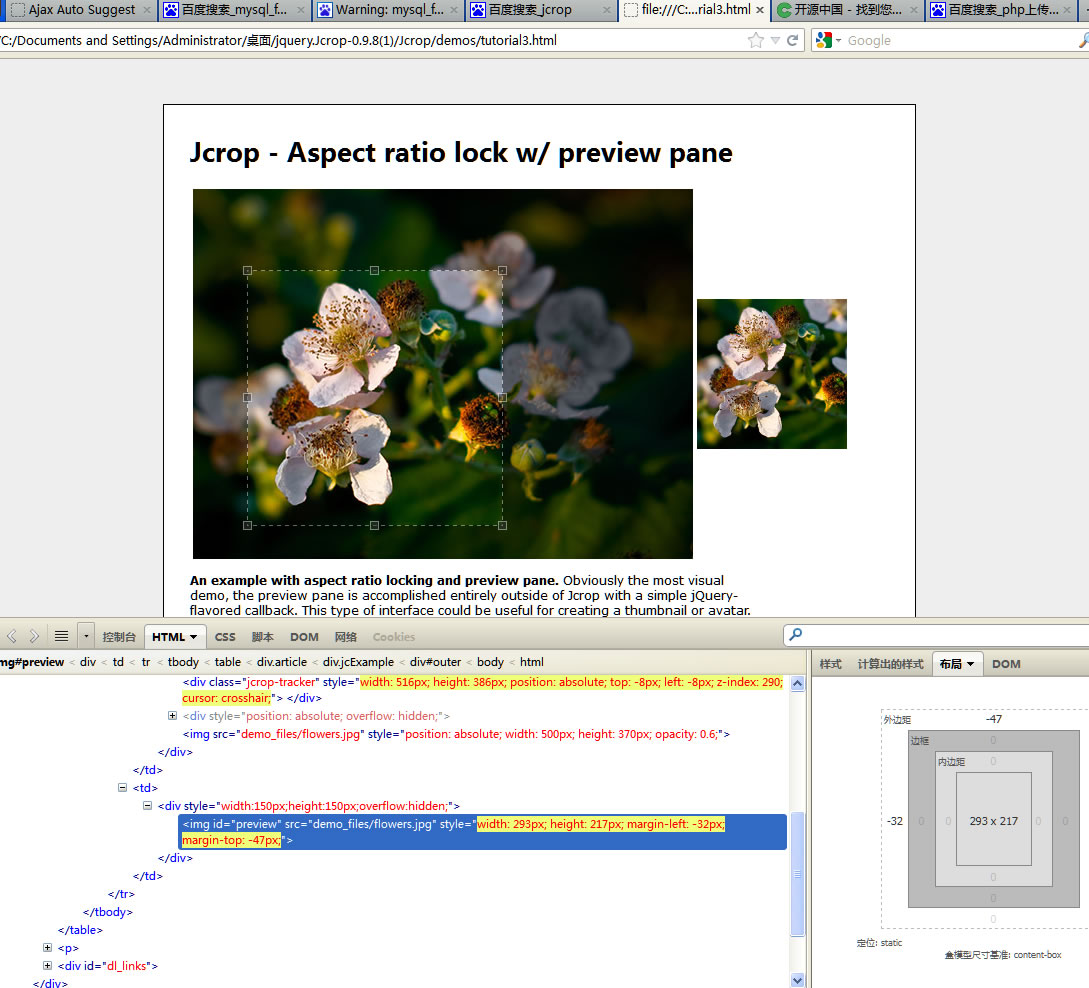0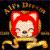0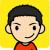jquery的 jcrop插件吧。。。js负责不了裁剪图片，只是把裁剪尺寸给了后端。。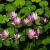0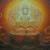00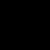0list(\$src_w,\$src_h)=getimagesize(\$src_img);  // 获取原图尺寸

\$dst_scale = \$dst_h/\$dst_w; //目标图像长宽比
\$src_scale = \$src_h/\$src_w; // 原图长宽比

if(\$src_scale>=\$dst_scale){  // 过高
\$w = intval(\$src_w);
\$h = intval(\$dst_scale*\$w);

\$x = 0;
\$y = (\$src_h - \$h)/3;
}
else{ // 过宽
\$h = intval(\$src_h);
\$w = intval(\$h/\$dst_scale);

\$x = (\$src_w - \$w)/2;
\$y = 0;
}

// 剪裁
\$source=imagecreatefromjpeg(\$src_img);
\$croped=imagecreatetruecolor(\$w, \$h);
imagecopy(\$croped,\$source,0,0,\$x,\$y,\$src_w,\$src_h);

// 缩放
\$scale = \$dst_w/\$w;
\$target = imagecreatetruecolor(\$dst_w, \$dst_h);
\$final_w = intval(\$w*\$scale);
\$final_h = intval(\$h*\$scale);
imagecopyresampled(\$target,\$croped,0,0,0,0,\$final_w,\$final_h,\$w,\$h);

// 保存
\$timestamp = time();
imagejpeg(\$target, "\$timestamp.jpg");
imagedestroy(\$target);

0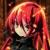PS:python有opencv库哦，图像处理很方便的。

0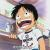PHP简单啊 把 jcrop提交过去的坐标跟大小 用PHP剪裁下就可以了